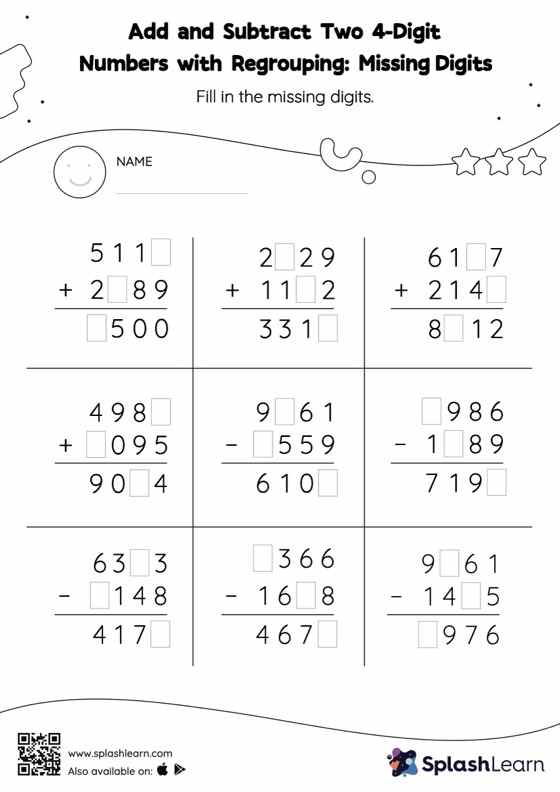# Add and Subtract Two 4-Digit Numbers with Regrouping: Missing Digits Worksheet

Home > Add and Subtract Two 4-Digit Numbers with Regrouping: Missing DigitsThis worksheet invites students to add and subtract two 4-digit numbers with regrouping, which helps them become proficient in addition and subtraction. In this worksheet, students practice solving problems using the column method. This method is especially helpful with problems involving bigger multi-digit numbers as the format provides an easy structure to follow.